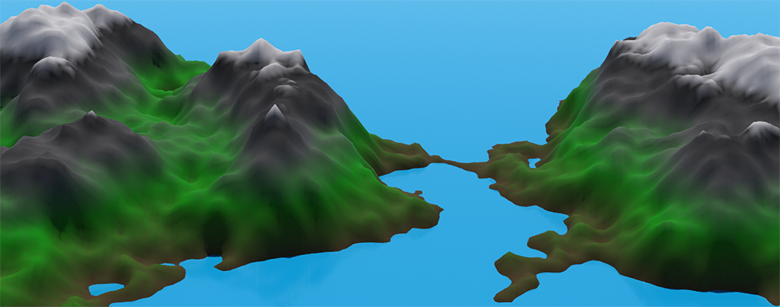# Daily #1: Diamond-Square

Inspired by my internet heroes, Mike Winkelmann and Sean “Day” Plott, my goal for this year is to produce a daily bit of content to help keep me practiced and showcase my skills. I’d rather not call it a “resolution” per se, since we all know what happens to those… So without any further ado:

Today’s daily involves learning about and implementing the diamond-square algorithm in Side Effects Software’s Houdini’s VEX language. In a nutshell, the algorithm performs a repeated sequence of steps that generate a heightmap from values seeded at the 4 corners of a grid. The result mimics a realistic looking landscapes quite well and can be used to generate procedural terrain.

The first step in the algorithm ‘diamonds’ the corner values into the middle one by averaging them and then adding a random offset. ‘Squaring’ is performed on the midpoints between each corner, where the adjacent values are averaged and offset. The grid is subdivided into grids of half the size, and then the algorithm is repeated. Using a grid of sides 2n + 1 in length ensures this can be performed a fixed number of times while hitting every point.

There are quite a few sites detailing the implementation, including the original paper, but I found Hunter Loftis’ version concise and easy to follow. I did the (trivially) easy job of translating it into VEX and applied it via a wrangle SOP to a grid with a 2n+1 side length. This quick and dirty version has a few caveats, such as relying on VEX array accessors to return 0 when out of bounds. One interesting thing I ran into was that it appears you can’t read and write (use `point` and `setpointattrib`) on the same geometry handle - all my values came out 0 when reading a written to attribute. From a threading perspective, this makes sense, but I may be wrong so it’s something I’m going to look into.

The following is the VEX code used:

``````int gridSize = chi("../grid1/rows"); // Assume 2n+1
float roughness = chf("roughness");

float heightMap[];
resize(heightMap, gridSize * gridSize);

int size = gridSize - 1;
int half = size / 2;
float scale = roughness * size;

// Initialize corner values, zero in this case
heightMap[0 + 0 * gridSize] = 0;
heightMap[(gridSize - 1) + 0 * gridSize] = 0;
heightMap[0 + (gridSize - 1) * gridSize] = 0;
heightMap[(gridSize - 1) + (gridSize - 1) * gridSize] = 0;

while (half >= 1)
{
// Diamond step
for (int y = half; y <= gridSize - 1; y += size)
{
for (int x = half; x <= gridSize - 1; x += size)
{
float a = heightMap[(x - half) + (y - half) * gridSize];
float b = heightMap[(x + half) + (y - half) * gridSize];
float c = heightMap[(x - half) + (y + half) * gridSize];
float d = heightMap[(x + half) + (y + half) * gridSize];

heightMap[x + y * gridSize] = (a + b + c + d) / 4.0 + (nrandom() * scale * 2.0 - scale);
}
}

// Square step
for (int y = 0; y <= gridSize - 1; y += half)
{
for (int x = (y + half) % size; x <= gridSize - 1; x += size)
{
float a = heightMap[ x             + (y - half) * gridSize];
float b = heightMap[ x             + (y + half) * gridSize];
float c = heightMap[(x - half) +  y             * gridSize];
float d = heightMap[(x + half) +  y             * gridSize];

heightMap[x + y * gridSize] = (a + b + c + d) / 4.0 + (nrandom() * scale * 2.0 - scale);
}
}

size = size / 2;
half = size / 2;
scale = roughness * size;
}

// Apply heightmap to grid points
for (int y = 0; y < gridSize; ++y)
{
for (int x = 0; x < gridSize; ++x)
{
setpointattrib(geoself(), "height", x + y * gridSize, heightMap[x + y * gridSize]);
}
}
``````

Using the height attribute to adjust y-position, and slapping a color SOP ramped to the height attribute, produced the following randomly generated terrain:Updated: